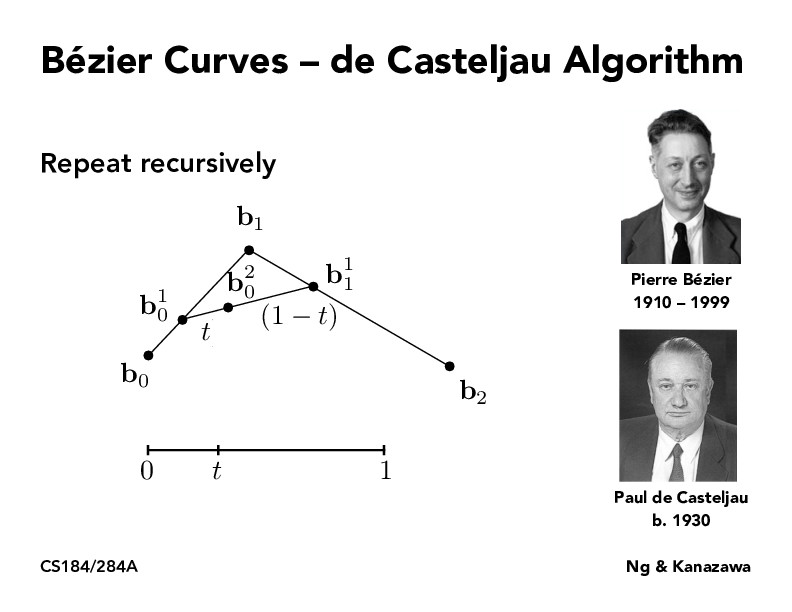Lecture 7: Splines and Bezier Curves (63)seohyunjeon

not 100% sure if i'm understanding the algorithm correctly but here goes nothing (please correct me if i am misunderstanding something!) we take a point that is some proportion t away from b0 and b1, and connect them as our "first degree" (superscript 1) points and connect them. We then take the point that is the same proportion t away from b_0^1 on our new line and conclude that that point is on the bezier curve. I assume if we were to implement this algorithm the smoothness of the bezier curve would be determined by how small we increment our t proportions by; what is considered an acceptably small increment of t to produce a smooth curve?

You must be enrolled in the course to comment